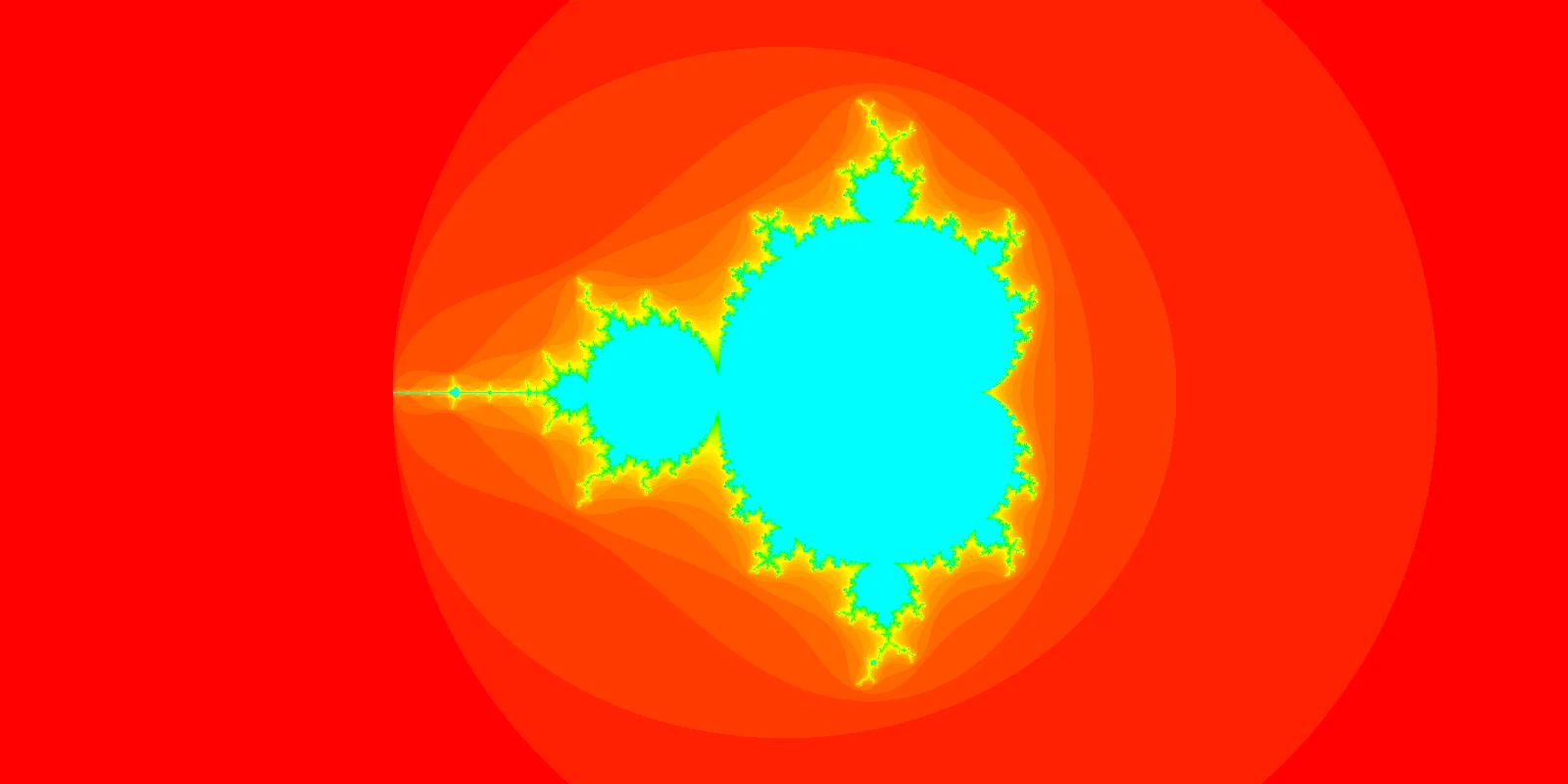The Python 🐍 problem-solving bootcamp 🚀 is starting soon. Join the second cohort now!

## Fractals and the Mandelbrot set

I have always liked the concept of fractal. They are very beautiful, they have a notion of infinity embedded in them, and they make no sense (seriously though, self-similarity?). How could they not be loved?

# Fractals and the Mandelbrot setDespite being fond of fractals, I had never understood them because I didn't know how to mathematically define one. I knew how to draw some, for example the snowflake or the Sierpinski triangle, but drawing a fractal and mathematically defining it aren't quite the same thing...

Enlightenment struck after watching this video from Numberphile about the Mandelbrot set, the fractal I presented above. After all, a fractal like this was not but a simple formula and a check for a bound on a sequence! I recommend to all of you that you watch that video.

To decide if a complex number $$c$$ belongs to the fractal or not, you define $$f(x) = x^2 + c$$ and then you look at the sequence $$c, f(c), f(f(c)), f(f(f(c))), ...$$. If the absolute value of these numbers stays bounded, then $$c$$ belongs to the Mandelbrot set. If these numbers keep on growing in absolute value, then $$c$$ does not belong to the set.

After learning how to create the Mandelbrot set I put that knowledge to practice, making use of Python.

The script below uses pygame to create a simple rendering of the Mandelbrot set. The image will be black and white and you need to use Ctrl + C to stop the script after you're done looking at the image.

import pygame

WIDTH = HEIGHT = 400

screen = pygame.display.set_mode((WIDTH, HEIGHT))

def f(z, c):
return z**2 + c

MAX_ITER = 50
for x in range(WIDTH):
for y in range(HEIGHT):
re = 3 * x / WIDTH - 2.3
im = 3 * y / HEIGHT - 1.5
z = c = complex(re, im)
iter = 0
while iter < MAX_ITER and abs(z) < 2:
z = f(z, c)
iter += 1

if abs(z) < 2:
screen.set_at((x, y), pygame.Color(0, 0, 0))
else:
screen.set_at((x, y), pygame.Color(255, 255, 255))

pygame.display.flip()
pygame.image.save(screen, "bw.png")

while True:
for ev in pygame.event.get():
pass

This will open said pygame window and it will save an image bw.png with the rendering:

To make this slightly more interesting, we can create a list with shades of gray and assign each shade to an iteration number, so that the colour a pixel is coloured in depends on how quickly the while loop was broken. To achieve this, we need to modify the middle section of the code:

# We added this.
GRAYS = list(
reversed(
[pygame.Color(*(int(255 * i / MAX_ITER),) * 3) for i in range(MAX_ITER + 1)]
)
)

for x in range(WIDTH):
for y in range(HEIGHT):
re = 3 * x / WIDTH - 2.3
im = 3 * y / HEIGHT - 1.5
z = c = complex(re, im)
iter = 0
while iter < MAX_ITER and abs(z) < 2:
z = f(z, c)
iter += 1

screen.set_at((x, y), GRAYS[iter])  # <-- Modified here.

pygame.image.save(screen, "gray.png")  # <-- Save with a new name.

This produces the following rendering:

To make this even more colourful, we can do all sorts of crazy things. I played around with lots of lists of colours and I ended up finding this one that I like:

colours = []
for i in range(MAX_ITER + 1):
c = pygame.Color(0, 0, 0)  # Irrelevant RGB values.
c.hsla = (180 * (i / MAX_ITER) ** 0.8, 100, 50, 100)
colours.append(c)

This palette produces the much more colourful version you can find below:

Here is the full code, with the dimensions used to generate the thumbnail for this article:

import pygame

WIDTH = 1600
HEIGHT = 800

screen = pygame.display.set_mode((WIDTH, HEIGHT))

def f(z, c):
return z**2 + c

MAX_ITER = 50

# GRAYS = list(
#     reversed(
#         [pygame.Color(*(int(255 * i / MAX_ITER),) * 3) for i in range(MAX_ITER + 1)]
#     )
# )

colours = []
for i in range(MAX_ITER + 1):
c = pygame.Color(0, 0, 0)  # Create a colour instance.
c.hsla = (180 * (i / MAX_ITER) ** 0.8, 100, 50, 100)
colours.append(c)

for x in range(WIDTH):
for y in range(HEIGHT):
re = 6 * x / WIDTH - 3.5
im = 3 * y / HEIGHT - 1.5
z = c = complex(re, im)
iter = 0
while iter < MAX_ITER and abs(z) < 2:
z = f(z, c)
iter += 1

screen.set_at((x, y), colours[iter])

pygame.display.flip()
pygame.image.save(screen, "thumbnail.png")

while True:
for ev in pygame.event.get():
pass

## Next steps

There are plenty of things you can do if you want to keep exploring this world. Some ideas include: